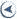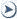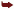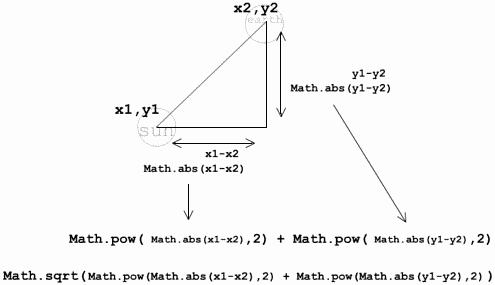# Workshop Chapter 12. Developing Time-Based AnimationsCONTENTS## Workshop Chapter 12. Developing Time-Based Animations

Flash is a frame-based animation tool. As you know, just because you set the frame rate to 120 frames per second, that doesn't mean Flash will really display that many frames in one second. The frame rate you specify is more of a top end that Flash will not exceed. Even if you keep your frame rate down in the normal range of 20 fps, there's a good chance that Flash will occasionally take longer than one-twentieth of a second to display a frame. The standard practice is to just make sure that your movie performs adequately on a slow machine, although there's still no guarantee.

If you use audio set to stream, Flash will drop frames to maintain a constant rate of sound. However, this approach to maintaining a particular speed has its drawbacks as well. This workshop chapter explores ways you can write your own code that effectively drops frames to keep up with a predetermined rate. This doesn't mean that you can actually reach the mythical 120 fps (Flash's maximum), but rather that you can make sure that your graphics stay on time. For example, you can designate that a circle will rotate fully in 10 seconds. On a super-slow machine, the circle might display only four times: at 0, 90, 180, and 360 degrees. On a fast machine, however, the circle might display at all 360 discrete angles. Regardless of the machine speed, however, one second after the clip starts, the circle will have made a full revolution. You'll learn how to accomplish this feat in this workshop chapter.

We're actually going to make circles rotate as well as revolve around other circles. The techniques learned, however, can also be applied to animations that aren't so predictable and geometric. For example, in Workshop Chapter 14, "Offline Production," you'll first learn how to collect data for any kind of animation offline and then apply the time-based animation knowledge from this workshop chapter.

This workshop chapter starts our animation efforts with circles:

1. Start a new movie and set the frame rate to 24 fps.

2. Draw a circle that will become a graphic of the earth. Make sure that you include additional graphical elements that will enable you to see when this graphic rotates (see Figure W12.1).

##### Figure W12.1. Draw some shapes in the "earth" circle so that you'll notice when the circle rotates.3. Convert the circle to a Movie Clip and name the instance earth. Place the following code in the first frame of the Movie Clip (where you'll put all the code for this workshop chapter):

`1 rpm = 60;  2 degreesPerSecond = (rpm*360)/60;  3 fps=24;  4 degreesPerFrame=degreesPerSecond/fps;  5 earth.onEnterFrame=function(){ 6   this._rotation += degreesPerFrame;  7 }`

This code isn't going to exhibit perfect synchronization. The rpm variable in line 1 is hard-wired to the number of times per minute the clip should rotate. We will use this variable to specify how fast, based on time, this clip should rotate. Line 2 translates revolutions per minute into the number of degreesPerSecond. Lines 3 and 4 become problematic. First, hard-wiring fps is going to prove to be a hassle and anyway, this movie should play the same regardless of the frame rate we specify. Line 4 accurately calculates the number of degreesPerFrame, but bases its calculation on fps, which could easily slow down. Finally, the onEnterFrame callback makes perfect sense provided that it is indeed executed 24 times a second.

## //

You can prove that this code doesn't work by first testing it and getting a feel for what 60 rpm looks like on your machine. Then change both the movie's frame rate and the fps value to, say, 120. The movie won't play quite as fast unless you have a really fast computer. This approach, which simply adds to _rotation in every enterFrame event, assumes that each frame is entered on time.

4. Change the onEnterFrame callback to read as follows:

`earth.onEnterFrame=function(){   now=getTimer();    this._rotation+=degreesPerSecond/(1000/(now-lastTime));    lastTime=now;  }`

The first thing you may notice is that because the variables fps and degreesPerFrame are no longer used, only the first two (of the four) lines that appear before the onEnterFrame callback are needed. Within the onEnterFrame callback, we first put the current getTimer() in a variable called now. Then we add the result of an expression to _rotation. Try the slow motion what-if possibility that we're supposed to rotate only 10 degrees per second: If it's been two seconds since the last time we rotated, we should rotate 20 degrees. If it's been only 0.5 second since we rotated, we need to rotate just 5 degrees. To calculate how long it's been since the last time we rotated, we subtract lastTime from now. The formula works like this: If lastTime was five past the hour and now is six past the hour, we know that one minute has elapsed but we're using milliseconds. If we divide 1000 into the number of milliseconds that have elapsed, we know how many seconds have passed. Then we divide that value into the number of degrees per second. (I think this formula is easiest to see when you try a few numbers.) Anyway, after we set the _rotation, we can save the current now into the variable lastTime so that the next time around we can calculate how long it's been since the last rotation.

It should work really well. Try changing the movie's frame rate to 10 fps or 90 fps. The movie might play smoother or clunkier, but it always stays "on time."

5. We can extend this workshop to practice both our math and our skills with time-based animation. Draw another circle to represent the sun. Convert it to a Movie clip and name the instance sun.

6. Before we change the onEnterFrame callback, we need to come up with different variable names. For example, if we want the earth to both rotate on its axis and revolve around the sun, the variable rpm could be confusing. (Is it "revolutions per minute" or "rotations per minute?") In any event, we'll need two variables, so start over with the following initialization script. (We'll do the onEnterFrame callback next.)

` 1 rotationsPerMinute = 60;   2 degreesPerSecond = (rotationsPerMinute*360)/60;   3 revolutionsPerMinute=20;   4 radiansPerSecond=(revolutionsPerMinute*Math.PI*2)/60;   5 orbitRadius=Math.sqrt(Math.pow ( Math.abs(sun._x-earth._x),2) + Math.pow ( Math.abs(sun._y-earth._y), 2 ))`

Notice that the first two lines are the same as before, but I changed rpm to rotationsPerMinute. Lines 3 5 set up the variables for positioning earth along a path. After hard-wiring a desired revolution speed (in line 3), radiansPerSecond is calculated. This is really the same as calculating degreesPerSecond; however, because I expect to use radians, I consider one revolution to be Math.PI*2 (rather than 360 as in the case of degrees). Finally, line 5 calculates the distance between the sun and earth clips (that is, the radius) using a formula based on Pythagoras' theorem.By the way, you can use the following handy-dandy formula any time you want to calculate the distance between two points: `Math.sqrt(Math.pow(Math.abs(x1-x2),2)+Math.pow(Math.abs(y1-y2),2 ));` In this syntax, x1 and y1 are the coordinates of one point, and x2 and y2 are the coordinates of the other point. For those of you who were awake in high-school Math class, this formula is based on Pythagoras' theorem (which, yes, does come up in everyday life). Pythagoras said that with a right triangle, the square of the length of one side added to the square of the length of the other side will equal the square of the third side. For any two points, we can easily find the difference in x and the difference in y so that we can draw the straight sides of a triangle. We can then raise those values to the second power (that is, square them), and then add them together. Finally, we know that that sum is the square of the diagonal line connecting the two points; when we take the square root of that sum, we end up with the actual length. Figure W12.2 shows how the formula is derived. Notice that when finding the difference between two points, I use Math.abs() to ensure that the value is not negative. It turns out that this shouldn't matter because when you square a negative number, the result should be positive. I'm leaving in that formula, however, because it always shows distance as a positive number.
##### Figure W12.2. Based on Pythagoras'theorem, this diagram shows you how to determine the distance between any two points.7. Now replace the onEnterFrame script:

` 1 earth.onEnterFrame=function(){  2   now=getTimer();   3   this._rotation+=degreesPerSecond/(1000/(now-lastTime));   4   5   radians= lastRad+radiansPerSecond/(1000/(now-lastTime));   6   this._x = sun._x+Math.cos(radians)* orbitRadius;   7   this._y = sun._y+Math.sin(radians)* orbitRadius;   8   9   lastRad=radians;  10   lastTime=now;  11 }`

Lines 1 3 are the same only the variable names have been changed. To understand line 5, realize that in line 3 we say "add to rotation" and we want to effectively say "add this many radians to the angle used to position the earth on its orbit." The earth instance doesn't have a "radians" property, however, and adding involves knowing where the earth instance was the previous time. That's why we add to lastRad (the variable assigned in line 9 to hold the last time around). Imagine if you were responsible for positioning the hour hand on a clock one hour ahead (that is, 1/12 of a circle more) you'd have to know where the hand was when you started (just like our code needs to know where the earth instance was positioned the last time). Once we know at what angle (in radians) the earth instance should be positioned, we use lines 6 and 7 to assign the _x and _y properties accordingly. (These two lines are just formulas used in Workshop Chapter 11, "Using Math to Create a Circular Slider.")

The result is pretty neat. Notice that you can position the earth instance in any location, and it will determine the circular path to follow based on a circle drawn with its center at the sun instance and its radius equal to the distance between sun and earth instances. Also notice that you can change the frame rate to anything you want, and the movie will always keep perfect synchronization (although the movie is sometimes jumpy when the frame rate is very low). You can also change the values for rotationsPerMinute and revolutionsPerMinute to effect how it rotates and revolves.

We have successfully created an animation that is based entirely on time, not on frame rate. Originally, I thought this exercise would be perfect for setInterval(); however, I'm not so sure that it makes much difference.

8. To see how you can use setInterval() as an alternative, first change the onEnterFrame callback to look like a function that is, so that the first line reads

`function update(){.`

In addition, change the three cases of this to earth.

9. Finally, add the following code somewhere outside the function:

`setInterval(update,50);`

You can change the 50 to anything you want. There really isn't much difference in this approach. If, however, you really believe that setInterval will trigger the update() function every 1/20th of a second (based on 50 milliseconds) without fail (which it won't), you can forgo most of the code we came up with in this workshop. That is, you could have something as simple as this version:

`function update(frequency){   earth._rotation+=degreesPerSecond/(1000/frequency);    radians= lastRad+radiansPerSecond/(1000/frequency);    earth._x = sun._x+Math.cos(radians)* orbitRadius;    earth._y = sun._y+Math.sin(radians)* orbitRadius;    lastRad=radians;  }  setInterval(update,50,50);`

First, notice that anything to do with getTimer() is gone (including the now variable). In place of (lastTime=now), I just use the parameter frequency, which contains how frequently this function is being called. That is, the third parameter for setInterval() is a value that gets sent to the function it triggers. You can learn more about setInterval() in Chapter 14, "Extending ActionScript." Realize that the preceding code assumes setInterval() is working perfectly. A true time-based solution uses getTimer() to check to see how much time has elapsed since the last execution. Only this last bit of code (after step 9) exhibits a non time-based solution.

What if you want to make an animation that's not based on math, but you still want to make the animation time-based? We'll do that in Workshop Chapter 14, "Offline Production." In brief, there are two basic steps to making a time-based animation that's not based on math: First you gather the coordinates for where the graphic you're animating should be at key moments in time, and then you use those values in an enterFrame event that keeps checking getTimer(). I know that explanation isn't complete, but you'll see how it's done in Workshop Chapter 14.CONTENTSActionScripting in MacromediaR FlashT MX
ISBN: N/A
EAN: N/A
Year: 2001
Pages: 41